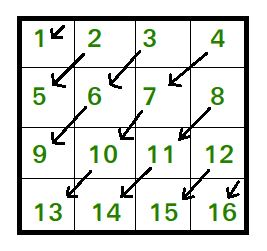Skip to content
Related Articles
Print the matrix diagonally downwards
• Difficulty Level : Easy
• Last Updated : 31 May, 2021

Given a matrix of size n*n, print the matrix in the following pattern.Output: 1 2 5 3 6 9 4 7 10 13 8 11 14 12 15 16
Examples:

```Input :matrix= { {1, 2},
{3, 4} }
Output : 1 2 3 4

Input :matrix= { {1, 2, 3},
{4, 5, 6},
{7, 8, 9} }
Output : 1 2 4 3 5 7 6 8 9```

Following is the C++ implementation for the above pattern.

## C++

 `// CPP program to print matrix downward``#include ``using` `namespace` `std;` `void` `printMatrixDiagonallyDown(vector > matrix,``                                                    ``int` `n)``{``    ``// printing elements above and on``    ``// second diagonal``    ``for` `(``int` `k = 0; k < n; k++) {` `        ``// traversing downwards starting``        ``// from first row``        ``int` `row = 0, col = k;``        ``while` `(col >= 0) {``            ``cout << matrix[row][col] << ``" "``;``            ``row++, col--;``        ``}``    ``}` `    ``// printing elements below second``    ``// diagonal``    ``for` `(``int` `j = 1; j < n; j++) {` `        ``// traversing downwards starting``        ``// from last column``        ``int` `col = n - 1, row = j;``        ``while` `(row < n) {``            ``cout << matrix[row][col] << ``" "``;``            ``row++, col--;``        ``}``    ``}``}` `int` `main()``{``    ``vector > matrix{ { 1, 2, 3 },``                                 ``{ 4, 5, 6 },``                                 ``{ 7, 8, 9 } };``    ``int` `n = 3;``    ``printMatrixDiagonallyDown(matrix, n);``    ``return` `0;``}`

## Java

 `// JAVA program to print``// matrix downward``class` `GFG{``static` `void` `printMatrixDiagonallyDown(``int``[][] matrix,``                                      ``int` `n)``{``  ``// printing elements above and on``  ``// second diagonal``  ``for` `(``int` `k = ``0``; k < n; k++)``  ``{``    ``// traversing downwards``    ``// starting from first row``    ``int` `row = ``0``, col = k;``    ``while` `(col >= ``0``)``    ``{``      ``System.out.print(matrix[row][col] + ``" "``);``      ``row++;``      ``col--;``    ``}``  ``}` `  ``// printing elements below``  ``// second diagonal``  ``for` `(``int` `j = ``1``; j < n; j++)``  ``{``    ``// traversing downwards starting``    ``// from last column``    ``int` `col = n - ``1``, row = j;``    ``while` `(row < n)``    ``{``      ``System.out.print(matrix[row][col] + ``" "``);``      ``row++;``      ``col--;``    ``}``  ``}``}` `// Driver code``public` `static` `void` `main(String[] args)``{``  ``int``[][] matrix = {{``1``, ``2``, ``3``},``                    ``{``4``, ``5``, ``6``},``                    ``{``7``, ``8``, ``9``}};``  ``int` `n = ``3``;``  ``printMatrixDiagonallyDown(matrix, n);``}``}` `// This code is contributed by Rajput-Ji`

## Python 3

 `# Python 3 program to print matrix downward` `def` `printMatrixDiagonallyDown(matrix,n):``    ``# printing elements above and on``    ``# second diagonal``    ``for` `k ``in` `range``(n):``        ``# traversing downwards starting``        ``# from first row``        ``row ``=` `0``        ``col ``=` `k``        ``while` `(col >``=` `0``):``            ``print``(matrix[row][col],end ``=` `" "``)``            ``row ``+``=` `1``            ``col ``-``=` `1` `    ``# printing elements below second``    ``# diagonal``    ``for` `j ``in` `range``(``1``,n):``        ``# traversing downwards starting``        ``# from last column``        ``col ``=` `n ``-` `1``        ``row ``=` `j``        ``while` `(row < n):``            ``print``(matrix[row][col],end ``=` `" "``)``            ``row ``+``=` `1``            ``col ``-``=` `1` `if` `__name__ ``=``=` `'__main__'``:``    ``matrix ``=` `[[``1``, ``2``, ``3``],[``4``, ``5``, ``6``],[``7``, ``8``, ``9``]]``    ``n ``=` `3``    ``printMatrixDiagonallyDown(matrix, n)` `# This code is contributed by Surendra_Gangwar`

## C#

 `// C# program to print``// matrix downward``using` `System;``class` `GFG{``static` `void` `printMatrixDiagonallyDown(``int``[,] matrix,``                                      ``int` `n)``{``  ``// printing elements above and on``  ``// second diagonal``  ``for` `(``int` `k = 0; k < n; k++)``  ``{``    ``// traversing downwards``    ``// starting from first row``    ``int` `row = 0, col = k;``    ` `    ``while` `(col >= 0)``    ``{``      ``Console.Write(matrix[row,col] + ``" "``);``      ``row++;``      ``col--;``    ``}``  ``}` `  ``// printing elements below``  ``// second diagonal``  ``for` `(``int` `j = 1; j < n; j++)``  ``{``    ``// traversing downwards starting``    ``// from last column``    ``int` `col = n - 1, row = j;``    ``while` `(row < n)``    ``{``      ``Console.Write(matrix[row,col] + ``" "``);``      ``row++;``      ``col--;``    ``}``  ``}``}` `// Driver code``public` `static` `void` `Main(String[] args)``{``  ``int``[,] matrix = {{1, 2, 3},``                   ``{4, 5, 6},``                   ``{7, 8, 9}};``  ``int` `n = 3;``  ``printMatrixDiagonallyDown(matrix, n);``}``}` `// This code is contributed by Amit Katiyar`

## Javascript

 ``

Output:

`1 2 4 3 5 7 6 8 9`

Time Complexity: O(n*n)

Attention reader! Don’t stop learning now. Get hold of all the important DSA concepts with the DSA Self Paced Course at a student-friendly price and become industry ready.  To complete your preparation from learning a language to DS Algo and many more,  please refer Complete Interview Preparation Course.

In case you wish to attend live classes with industry experts, please refer Geeks Classes Live

My Personal Notes arrow_drop_up